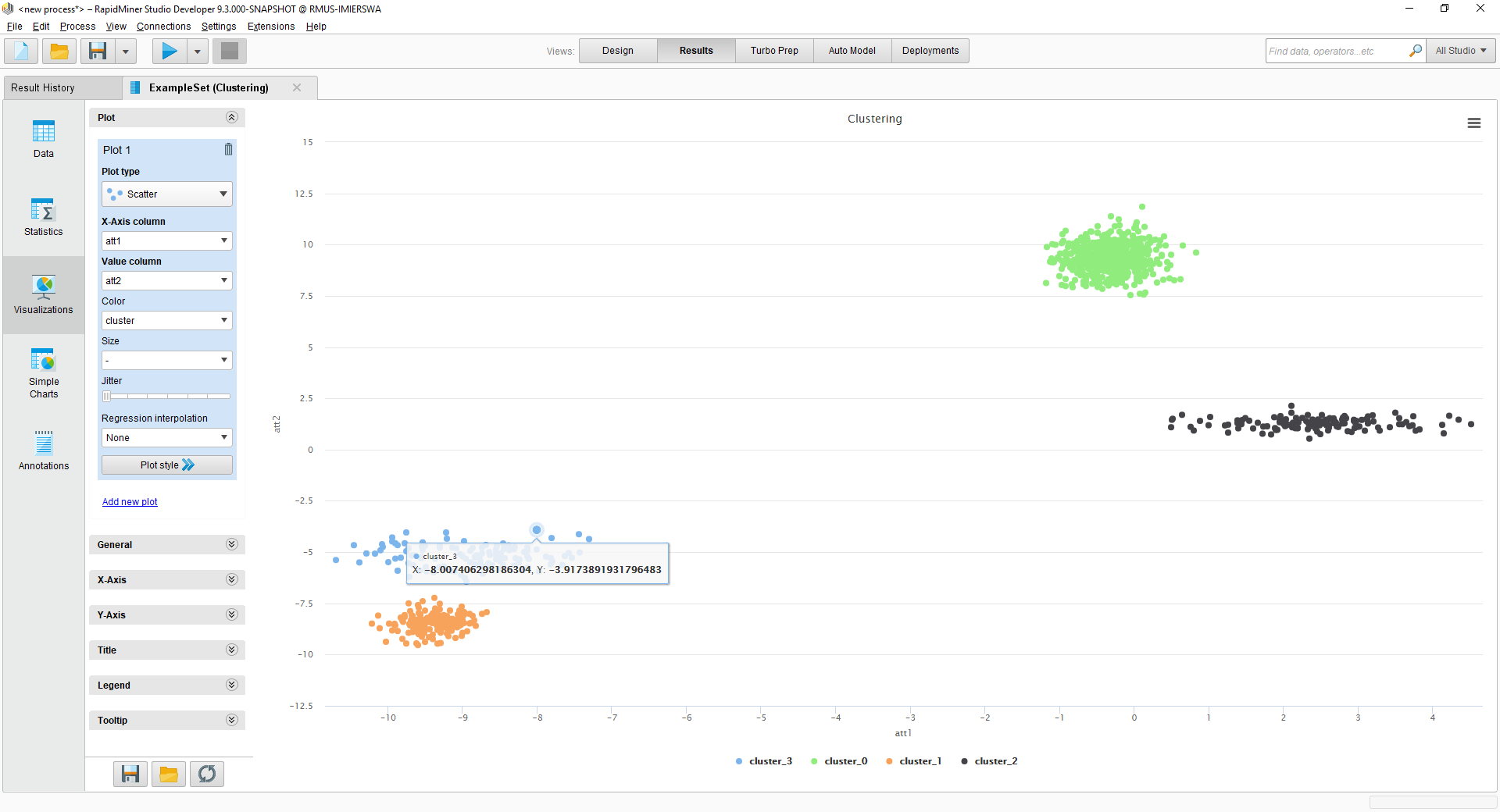# draw scatter plot for cluster using execute python

Member Posts: 16Contributor II
in Help
i used kmeans cluster operator to cluster two columns and i want to draw the scatter plot for the resulting clusters. i tried using executer python operator but its input parameters doesn't accept text as cluster. can you tell me the way i can do it please

• Administrator, Moderator, Employee, RapidMiner Certified Analyst, RapidMiner Certified Expert, Community Manager, RMResearcher, Member, University Professor Posts: 1,751RM Founder
Hi,
You really do not need to use Python for the plotting to be honest.  I have attached a process below which generates two columns and clusters the data.  At the end, you can simply click on Visualizations and set up the chart as desired (see below):The process is below.
Hope this helps,
Ingo

`<?xml version="1.0" encoding="UTF-8"?><process version="9.3.000-SNAPSHOT"><br>&nbsp; <context><br>&nbsp;&nbsp;&nbsp; <input/><br>&nbsp;&nbsp;&nbsp; <output/><br>&nbsp;&nbsp;&nbsp; <macros/><br>&nbsp; </context><br>&nbsp; <operator activated="true" class="process" compatibility="9.3.000-SNAPSHOT" expanded="true" name="Process"><br>&nbsp;&nbsp;&nbsp; <parameter key="logverbosity" value="init"/><br>&nbsp;&nbsp;&nbsp; <parameter key="random_seed" value="2001"/><br>&nbsp;&nbsp;&nbsp; <parameter key="send_mail" value="never"/><br>&nbsp;&nbsp;&nbsp; <parameter key="notification_email" value=""/><br>&nbsp;&nbsp;&nbsp; <parameter key="process_duration_for_mail" value="30"/><br>&nbsp;&nbsp;&nbsp; <parameter key="encoding" value="UTF-8"/><br>&nbsp;&nbsp;&nbsp; <process expanded="true"><br>&nbsp;&nbsp;&nbsp;&nbsp;&nbsp; <operator activated="true" class="generate_data" compatibility="9.3.000-SNAPSHOT" expanded="true" height="68" name="Generate Data" width="90" x="45" y="34"><br>&nbsp;&nbsp;&nbsp;&nbsp;&nbsp;&nbsp;&nbsp; <parameter key="target_function" value="gaussian mixture clusters"/><br>&nbsp;&nbsp;&nbsp;&nbsp;&nbsp;&nbsp;&nbsp; <parameter key="number_examples" value="1000"/><br>&nbsp;&nbsp;&nbsp;&nbsp;&nbsp;&nbsp;&nbsp; <parameter key="number_of_attributes" value="2"/><br>&nbsp;&nbsp;&nbsp;&nbsp;&nbsp;&nbsp;&nbsp; <parameter key="attributes_lower_bound" value="-10.0"/><br>&nbsp;&nbsp;&nbsp;&nbsp;&nbsp;&nbsp;&nbsp; <parameter key="attributes_upper_bound" value="10.0"/><br>&nbsp;&nbsp;&nbsp;&nbsp;&nbsp;&nbsp;&nbsp; <parameter key="gaussian_standard_deviation" value="10.0"/><br>&nbsp;&nbsp;&nbsp;&nbsp;&nbsp;&nbsp;&nbsp; <parameter key="largest_radius" value="10.0"/><br>&nbsp;&nbsp;&nbsp;&nbsp;&nbsp;&nbsp;&nbsp; <parameter key="use_local_random_seed" value="false"/><br>&nbsp;&nbsp;&nbsp;&nbsp;&nbsp;&nbsp;&nbsp; <parameter key="local_random_seed" value="1992"/><br>&nbsp;&nbsp;&nbsp;&nbsp;&nbsp;&nbsp;&nbsp; <parameter key="datamanagement" value="double_array"/><br>&nbsp;&nbsp;&nbsp;&nbsp;&nbsp;&nbsp;&nbsp; <parameter key="data_management" value="auto"/><br>&nbsp;&nbsp;&nbsp;&nbsp;&nbsp; </operator><br>&nbsp;&nbsp;&nbsp;&nbsp;&nbsp; <operator activated="true" class="select_attributes" compatibility="9.3.000-SNAPSHOT" expanded="true" height="82" name="Select Attributes" width="90" x="179" y="34"><br>&nbsp;&nbsp;&nbsp;&nbsp;&nbsp;&nbsp;&nbsp; <parameter key="attribute_filter_type" value="single"/><br>&nbsp;&nbsp;&nbsp;&nbsp;&nbsp;&nbsp;&nbsp; <parameter key="attribute" value="label"/><br>&nbsp;&nbsp;&nbsp;&nbsp;&nbsp;&nbsp;&nbsp; <parameter key="attributes" value=""/><br>&nbsp;&nbsp;&nbsp;&nbsp;&nbsp;&nbsp;&nbsp; <parameter key="use_except_expression" value="false"/><br>&nbsp;&nbsp;&nbsp;&nbsp;&nbsp;&nbsp;&nbsp; <parameter key="value_type" value="attribute_value"/><br>&nbsp;&nbsp;&nbsp;&nbsp;&nbsp;&nbsp;&nbsp; <parameter key="use_value_type_exception" value="false"/><br>&nbsp;&nbsp;&nbsp;&nbsp;&nbsp;&nbsp;&nbsp; <parameter key="except_value_type" value="time"/><br>&nbsp;&nbsp;&nbsp;&nbsp;&nbsp;&nbsp;&nbsp; <parameter key="block_type" value="attribute_block"/><br>&nbsp;&nbsp;&nbsp;&nbsp;&nbsp;&nbsp;&nbsp; <parameter key="use_block_type_exception" value="false"/><br>&nbsp;&nbsp;&nbsp;&nbsp;&nbsp;&nbsp;&nbsp; <parameter key="except_block_type" value="value_matrix_row_start"/><br>&nbsp;&nbsp;&nbsp;&nbsp;&nbsp;&nbsp;&nbsp; <parameter key="invert_selection" value="true"/><br>&nbsp;&nbsp;&nbsp;&nbsp;&nbsp;&nbsp;&nbsp; <parameter key="include_special_attributes" value="true"/><br>&nbsp;&nbsp;&nbsp;&nbsp;&nbsp; </operator><br>&nbsp;&nbsp;&nbsp;&nbsp;&nbsp; <operator activated="true" class="concurrency:k_means" compatibility="9.3.000-SNAPSHOT" expanded="true" height="82" name="Clustering" width="90" x="313" y="34"><br>&nbsp;&nbsp;&nbsp;&nbsp;&nbsp;&nbsp;&nbsp; <parameter key="add_cluster_attribute" value="true"/><br>&nbsp;&nbsp;&nbsp;&nbsp;&nbsp;&nbsp;&nbsp; <parameter key="add_as_label" value="false"/><br>&nbsp;&nbsp;&nbsp;&nbsp;&nbsp;&nbsp;&nbsp; <parameter key="remove_unlabeled" value="false"/><br>&nbsp;&nbsp;&nbsp;&nbsp;&nbsp;&nbsp;&nbsp; <parameter key="k" value="4"/><br>&nbsp;&nbsp;&nbsp;&nbsp;&nbsp;&nbsp;&nbsp; <parameter key="max_runs" value="10"/><br>&nbsp;&nbsp;&nbsp;&nbsp;&nbsp;&nbsp;&nbsp; <parameter key="determine_good_start_values" value="true"/><br>&nbsp;&nbsp;&nbsp;&nbsp;&nbsp;&nbsp;&nbsp; <parameter key="measure_types" value="BregmanDivergences"/><br>&nbsp;&nbsp;&nbsp;&nbsp;&nbsp;&nbsp;&nbsp; <parameter key="mixed_measure" value="MixedEuclideanDistance"/><br>&nbsp;&nbsp;&nbsp;&nbsp;&nbsp;&nbsp;&nbsp; <parameter key="nominal_measure" value="NominalDistance"/><br>&nbsp;&nbsp;&nbsp;&nbsp;&nbsp;&nbsp;&nbsp; <parameter key="numerical_measure" value="EuclideanDistance"/><br>&nbsp;&nbsp;&nbsp;&nbsp;&nbsp;&nbsp;&nbsp; <parameter key="divergence" value="SquaredEuclideanDistance"/><br>&nbsp;&nbsp;&nbsp;&nbsp;&nbsp;&nbsp;&nbsp; <parameter key="kernel_type" value="radial"/><br>&nbsp;&nbsp;&nbsp;&nbsp;&nbsp;&nbsp;&nbsp; <parameter key="kernel_gamma" value="1.0"/><br>&nbsp;&nbsp;&nbsp;&nbsp;&nbsp;&nbsp;&nbsp; <parameter key="kernel_sigma1" value="1.0"/><br>&nbsp;&nbsp;&nbsp;&nbsp;&nbsp;&nbsp;&nbsp; <parameter key="kernel_sigma2" value="0.0"/><br>&nbsp;&nbsp;&nbsp;&nbsp;&nbsp;&nbsp;&nbsp; <parameter key="kernel_sigma3" value="2.0"/><br>&nbsp;&nbsp;&nbsp;&nbsp;&nbsp;&nbsp;&nbsp; <parameter key="kernel_degree" value="3.0"/><br>&nbsp;&nbsp;&nbsp;&nbsp;&nbsp;&nbsp;&nbsp; <parameter key="kernel_shift" value="1.0"/><br>&nbsp;&nbsp;&nbsp;&nbsp;&nbsp;&nbsp;&nbsp; <parameter key="kernel_a" value="1.0"/><br>&nbsp;&nbsp;&nbsp;&nbsp;&nbsp;&nbsp;&nbsp; <parameter key="kernel_b" value="0.0"/><br>&nbsp;&nbsp;&nbsp;&nbsp;&nbsp;&nbsp;&nbsp; <parameter key="max_optimization_steps" value="100"/><br>&nbsp;&nbsp;&nbsp;&nbsp;&nbsp;&nbsp;&nbsp; <parameter key="use_local_random_seed" value="false"/><br>&nbsp;&nbsp;&nbsp;&nbsp;&nbsp;&nbsp;&nbsp; <parameter key="local_random_seed" value="1992"/><br>&nbsp;&nbsp;&nbsp;&nbsp;&nbsp; </operator><br>&nbsp;&nbsp;&nbsp;&nbsp;&nbsp; <connect from_op="Generate Data" from_port="output" to_op="Select Attributes" to_port="example set input"/><br>&nbsp;&nbsp;&nbsp;&nbsp;&nbsp; <connect from_op="Select Attributes" from_port="example set output" to_op="Clustering" to_port="example set"/><br>&nbsp;&nbsp;&nbsp;&nbsp;&nbsp; <connect from_op="Clustering" from_port="clustered set" to_port="result 1"/><br>&nbsp;&nbsp;&nbsp;&nbsp;&nbsp; <portSpacing port="source_input 1" spacing="0"/><br>&nbsp;&nbsp;&nbsp;&nbsp;&nbsp; <portSpacing port="sink_result 1" spacing="0"/><br>&nbsp;&nbsp;&nbsp;&nbsp;&nbsp; <portSpacing port="sink_result 2" spacing="0"/><br>&nbsp;&nbsp;&nbsp; </process><br>&nbsp; </operator><br></process>`

• Member Posts: 16Contributor II
thank you so much
but i want to make 3 dimensional visualization, can i do it without using python code
• Administrator, Moderator, Employee, RapidMiner Certified Analyst, RapidMiner Certified Expert, Community Manager, RMResearcher, Member, University Professor Posts: 1,751RM Founder
Yes, that is possible as well.  You simply select "Scatter 3D" as the plot type.